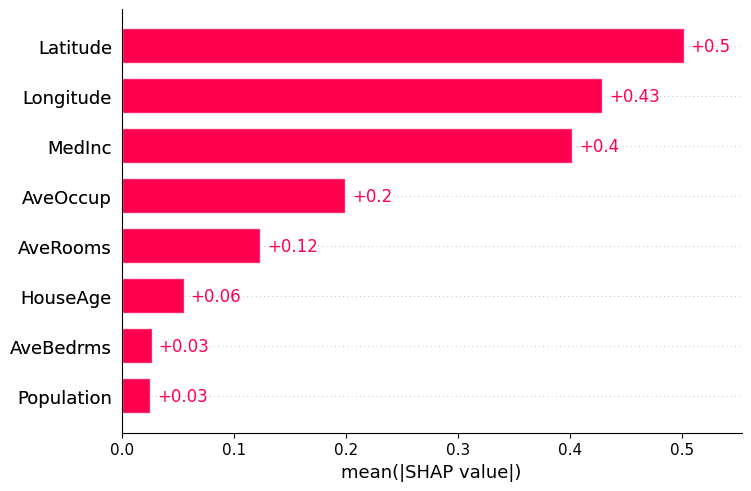# Front page example (XGBoost)

The code from the front page example using XGBoost.

:

import xgboost
import shap

# train XGBoost model
X,y = shap.datasets.boston()
model = xgboost.XGBRegressor(max_depth=1).fit(X, y)

# explain the model's predictions using SHAP values
# (same syntax works for LightGBM, CatBoost, and scikit-learn models)
shap_values = explainer(X)

# visualize the first prediction's explanation
shap.plots.waterfall(shap_values)

---------------------------------------------------------------------------
AttributeError                            Traceback (most recent call last)
<ipython-input-8-fa7a6360ae5e> in <module>
9 # (same syntax works for LightGBM, CatBoost, and scikit-learn models)
12 shap_values = explainer(X)
13

AttributeError: 'XGBRegressor' object has no attribute 'predict_proba'

:

import xgboost
import shap

# train XGBoost model
model = xgboost.XGBClassifier(max_depth=1, learning_rate=0.5).fit(X, y)

# explain the model's predictions using SHAP values
# (same syntax works for LightGBM, CatBoost, and scikit-learn models)
def f(x):
shap_values = explainer(X[:100])

# visualize the first prediction's explanation
shap.plots.waterfall(shap_values):

f(background.data).mean()

:

0.24263532

:

f(background.data).mean()

:

0.24263532

[ ]:



[ ]:



:

shap_values.expected_value

:

0.242635348305339

:

shap.plots.dependence(shap_values[:,"Age"]):

shap.plots.dependence(shap_values[:,"Age"]):

# plot the global importance of each feature
shap.plots.bar(shap_values):

shap.plots.initjs()

# visualize the first prediction's explanation
shap.plots.force(shap_values):

Visualization omitted, Javascript library not loaded!
Have you run initjs() in this notebook? If this notebook was from another user you must also trust this notebook (File -> Trust notebook). If you are viewing this notebook on github the Javascript has been stripped for security. If you are using JupyterLab this error is because a JupyterLab extension has not yet been written.
:

# visualize the first prediction's explanation
shap.plots.force(shap_values)

:

Visualization omitted, Javascript library not loaded!
Have you run initjs() in this notebook? If this notebook was from another user you must also trust this notebook (File -> Trust notebook). If you are viewing this notebook on github the Javascript has been stripped for security. If you are using JupyterLab this error is because a JupyterLab extension has not yet been written.
:

# plot the importance of a single feature across all samples
shap.plots.dependence(shap_values[:,"RM"], color=shap_values):

# plot the global importance of each feature
shap.plots.bar(shap_values):

# plot the distribution of importances for each feature over all samples
shap.plots.summary(shap_values)